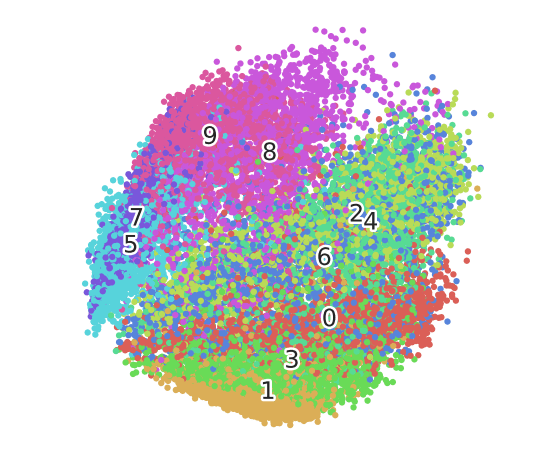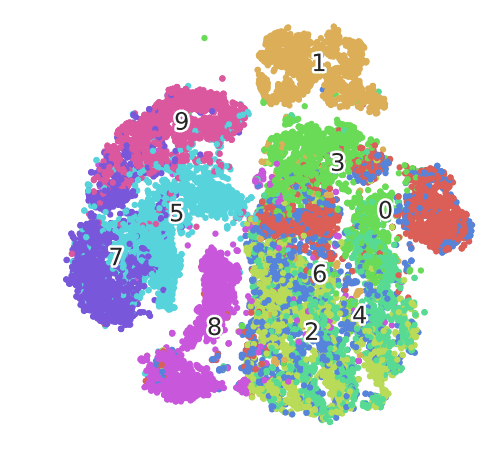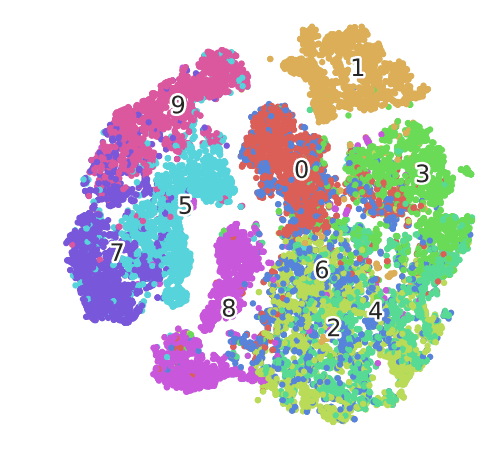Tutorials
data visualization
+1

# Introduction to t-SNE

In this tutorial, you’ll learn about the recently discovered Dimensionality Reduction technique known as t-Distributed Stochastic Neighbor Embedding (t-SNE).In this tutorial, you’ll learn about the recently discovered Dimensionality Reduction technique known as t-Distributed Stochastic Neighbor Embedding (t-SNE). More specifically you will :

• Learn about Dimensionality Reduction and its types.

• Learn about Principal Component Analysis (PCA) and its usage in python.

• Learn about t-Distributed Stochastic Neighbor Embedding (t-SNE) and its usage in python.

• Visualize the results of the two algorithms.

• Point out the differences between the two algorithms.

## Dimensionality Reduction

If you have worked with a dataset before with a lot of features, you can fathom how difficult it is to understand or explore the relationships between the features. Not only it makes the EDA process difficult but also affects the machine learning model’s performance since the chances are that you might overfit your model or violate some of the assumptions of the algorithm, like the independence of features in linear regression. This is where dimensionality reduction comes in. In machine learning, dimensionality reduction is the process of reducing the number of random variables under consideration by obtaining a set of principal variables. By reducing the dimension of your feature space, you have fewer relationships between features to consider which can be explored and visualized easily and also you are less likely to overfit your model.

Dimensionality reduction can be achieved in the following ways:

• Feature Elimination: You reduce the feature space by eliminating features. This has a disadvantage though, as you gain no information from those features that you have dropped.

• Feature Selection: You apply some statistical tests in order to rank them according to their importance and then select a subset of features for your work. This again suffers from information loss and is less stable as different test gives different importance score to features. You can check more on this here.

• Feature Extraction: You create new independent features, where each new independent feature is a combination of each of the old independent features. These techniques can further be divided into linear and non-linear dimensionality reduction techniques.

## Principal Component Analysis (PCA)

Principal Component Analysis or PCA is a linear feature extraction technique. It performs a linear mapping of the data to a lower-dimensional space in such a way that the variance of the data in the low-dimensional representation is maximized. It does so by calculating the eigenvectors from the covariance matrix. The eigenvectors that correspond to the largest eigenvalues (the principal components) are used to reconstruct a significant fraction of the variance of the original data.

In simpler terms, PCA combines your input features in a specific way that you can drop the least important feature while still retaining the most valuable parts of all of the features. As an added benefit, each of the new features or components created after PCA are all independent of one another.

## t-Distributed Stochastic Neighbor Embedding (t-SNE)

t-Distributed Stochastic Neighbor Embedding (t-SNE) is a non-linear technique for dimensionality reduction that is particularly well suited for the visualization of high-dimensional datasets. It is extensively applied in image processing, NLP, genomic data and speech processing. To keep things simple, here’s a brief overview of working of t-SNE:

• The algorithms starts by calculating the probability of similarity of points in high-dimensional space and calculating the probability of similarity of points in the corresponding low-dimensional space. The similarity of points is calculated as the conditional probability that a point A would choose point B as its neighbor if neighbors were picked in proportion to their probability density under a Gaussian (normal distribution) centered at A.
• It then tries to minimize the difference between these conditional probabilities (or similarities) in higher-dimensional and lower-dimensional space for a perfect representation of data points in lower-dimensional space.
• To measure the minimization of the sum of difference of conditional probability t-SNE minimizes the sum of Kullback-Leibler divergence of overall data points using a gradient descent method.

Note Kullback-Leibler divergence or KL divergence is is a measure of how one probability distribution diverges from a second, expected probability distribution.

Those who are interested in knowing the detailed working of an algorithm can refer to this research paper.

In simpler terms, t-Distributed stochastic neighbor embedding (t-SNE) minimizes the divergence between two distributions: a distribution that measures pairwise similarities of the input objects and a distribution that measures pairwise similarities of the corresponding low-dimensional points in the embedding.

In this way, t-SNE maps the multi-dimensional data to a lower dimensional space and attempts to find patterns in the data by identifying observed clusters based on similarity of data points with multiple features. However, after this process, the input features are no longer identifiable, and you cannot make any inference based only on the output of t-SNE. Hence it is mainly a data exploration and visualization technique.

## Using t-SNE in Python

Now you will apply t-SNE on an open source dataset and try to visualize the results. In addition, you will also visualize the output of PCA on the same dataset to compare it with that of t-SNE.

The dataset you will be using is Fashion-MNIST dataset and can be found here (don't forget to check it out!). The Fashion-MNIST dataset is a 28x28 grayscale image of 70,000 fashion products from 10 categories, with 7,000 images per category. The training set has 60,000 images, and the test set has 10,000 images. Fashion-MNIST is a replacement for the original MNIST dataset for producing better results, the image dimensions, training and test splits are similar to the original MNIST dataset. Similar to MNIST the Fashion-MNIST also consists of 10 labels, but instead of handwritten digits, you have 10 different labels of fashion accessories like sandals, shirt, trousers, etc.

Each training and test example is assigned to one of the following labels:

• 0 T-shirt/top
• 1 Trouser
• 2 Pullover
• 3 Dress
• 4 Coat
• 5 Sandal
• 6 Shirt
• 7 Sneaker
• 8 Bag
• 9 Ankle boot

You can find mnist_reader.py file which is present in utils folder of the Github repository. This file has a function load_mnist() which is specifically written to read .gz files, or you can define the function as follows:

# Fashion MNIST reader

import os
import gzip
import numpy as np

"""Load MNIST data from path"""
labels_path = os.path.join(path,
'%s-labels-idx1-ubyte.gz'
% kind)
images_path = os.path.join(path,
'%s-images-idx3-ubyte.gz'
% kind)

with gzip.open(labels_path, 'rb') as lbpath:
offset=8)

with gzip.open(images_path, 'rb') as imgpath:
offset=16).reshape(len(labels), 784)

return images, labels


You will apply the algorithms on the training set data. Use the load_mnist() function you just defined and pass the first argument as the location of the data files and second argument kind as 'train' to read the training set data.

X_train, y_train = load_mnist('Downloads/datasets/mnist/fashion_mnist', kind='train')


You can check the dimensions of the data using shape attribute. You will notice the training set has 60000 sample points and 784 features.

X_train.shape

(60000, 784)


The variable y_train contains the labels of every sample marked as integers from 0 to 9.

y_train

array([9, 0, 0, ..., 3, 0, 5], dtype=uint8)


Next, you will import the necessary libraries you will be using throughout the tutorial. To maintain reproducibility, you will define a random state variable RS and set it to 123.

import time
import pandas as pd
import numpy as np
import matplotlib.pyplot as plt
import matplotlib.patheffects as PathEffects
%matplotlib inline

import seaborn as sns
sns.set_style('darkgrid')
sns.set_palette('muted')
sns.set_context("notebook", font_scale=1.5,
rc={"lines.linewidth": 2.5})
RS = 123


In order to visualize the results of both the algorithms, you will create a fashion_scatter() function which takes two arguments: 1. x, which is a 2-D numpy array containing the output of the algorithm and 2. colors, which is 1-D numpy array containing the labels of the dataset. The function will render a scatter plot with as many unique colors as the number of classes in the variable colors.

# Utility function to visualize the outputs of PCA and t-SNE

def fashion_scatter(x, colors):
# choose a color palette with seaborn.
num_classes = len(np.unique(colors))
palette = np.array(sns.color_palette("hls", num_classes))

# create a scatter plot.
f = plt.figure(figsize=(8, 8))
ax = plt.subplot(aspect='equal')
sc = ax.scatter(x[:,0], x[:,1], lw=0, s=40, c=palette[colors.astype(np.int)])
plt.xlim(-25, 25)
plt.ylim(-25, 25)
ax.axis('off')
ax.axis('tight')

# add the labels for each digit corresponding to the label
txts = []

for i in range(num_classes):

# Position of each label at median of data points.

xtext, ytext = np.median(x[colors == i, :], axis=0)
txt = ax.text(xtext, ytext, str(i), fontsize=24)
txt.set_path_effects([
PathEffects.Stroke(linewidth=5, foreground="w"),
PathEffects.Normal()])
txts.append(txt)

return f, ax, sc, txts


To make sure you don’t burden your machine concerning memory and time you will only use the first 20,000 samples to run the algorithms on. Also, don't forget to check whether the first 20000 samples cover samples from all the 10 classes.

# Subset first 20k data points to visualize
x_subset = X_train[0:20000]
y_subset = y_train[0:20000]

print np.unique(y_subset)

[0 1 2 3 4 5 6 7 8 9]


Now you will use PCA on the subset data and visualize its output. You will use sklearn's PCA with n_components (Number of principal components to keep) equal to 4. There are a variety of parameters available in sklearn that can be tweaked, but for now, you will use default values. If you wish to know more about these parameters check out sklearn's PCA documentation.

from sklearn.decomposition import PCA

time_start = time.time()

pca = PCA(n_components=4)
pca_result = pca.fit_transform(x_subset)

print 'PCA done! Time elapsed: {} seconds'.format(time.time()-time_start)

PCA done! Time elapsed: 1.55137515068 seconds


Notice the time taken by PCA to run on x_subset with 20000 samples. Quite fast isn't it?

Now you will store all the four principal components in a new DataFrame pca_df and check the amount of variance of the data explained by these four components.

pca_df = pd.DataFrame(columns = ['pca1','pca2','pca3','pca4'])

pca_df['pca1'] = pca_result[:,0]
pca_df['pca2'] = pca_result[:,1]
pca_df['pca3'] = pca_result[:,2]
pca_df['pca4'] = pca_result[:,3]

print 'Variance explained per principal component: {}'.format(pca.explained_variance_ratio_)

Variance explained per principal component: [0.29021329 0.1778743  0.06015076 0.04975864]


Note that the first and second principal components explain almost 48% of the variance in the data x_subset. You will use these two components for visualization by passing them in the fashion_scatter() function.

top_two_comp = pca_df[['pca1','pca2']] # taking first and second principal component

fashion_scatter(top_two_comp.values,y_subset) # Visualizing the PCA output

(<matplotlib.figure.Figure at 0x7f380ee716d0>,
<matplotlib.axes._subplots.AxesSubplot at 0x7f380d56b710>,
<matplotlib.collections.PathCollection at 0x7f380eedec90>,
[Text(774.45,-689.695,u'0'),
Text(42.8638,-1429.36,u'1'),
Text(1098.28,376.975,u'2'),
Text(329.272,-1111.49,u'3'),
Text(1266.97,295.466,u'4'),
Text(-1589.56,61.8677,u'5'),
Text(713.473,-65.687,u'6'),
Text(-1521.41,335.666,u'7'),
Text(63.8177,1003.33,u'8'),
Text(-645.691,1168.24,u'9')])As you can notice, PCA has tried to separate the different points and form clustered groups of similar points. Also, the plot can be used for exploratory analysis. The principal components (pca1, pca2, etc.) can be used as features in classification or clustering algorithms.

Now you will do the same exercise using the t-SNE algorithm. Scikit-learn has an implementation of t-SNE available, and you can check its documentation here. It provides a wide variety of tuning parameters for t-SNE, and the most notable ones are:

• n_components (default: 2): Dimension of the embedded space.
• perplexity (default: 30): The perplexity is related to the number of nearest neighbors that are used in other manifold learning algorithms. Consider selecting a value between 5 and 50.
• early_exaggeration (default: 12.0): Controls how tight natural clusters in the original space are in the embedded space and how much space will be between them.
• learning_rate (default: 200.0): The learning rate for t-SNE is usually in the range (10.0, 1000.0).
• n_iter (default: 1000): Maximum number of iterations for the optimization. Should be at least 250.
• method (default: ‘barnes_hut’): Barnes-Hut approximation runs in O(NlogN) time. method=’exact’ will run on the slower, but exact, algorithm in O(N^2) time.

You will run t-SNE on x_subset with default parameters.

from sklearn.manifold import TSNE
import time
time_start = time.time()

fashion_tsne = TSNE(random_state=RS).fit_transform(x_subset)

print 't-SNE done! Time elapsed: {} seconds'.format(time.time()-time_start)

t-SNE done! Time elapsed: 1172.31058598 seconds


It can be seen that t-SNE takes considerably longer time to execute on the same sample size of data than PCA.

Visualizing the output of t-SNE using fashion_scatter() function:

fashion_scatter(fashion_tsne, y_subset)

(<matplotlib.figure.Figure at 0x7f380ee71410>,
<matplotlib.axes._subplots.AxesSubplot at 0x7f380ee92190>,
<matplotlib.collections.PathCollection at 0x7f384c0530d0>,
[Text(41.5947,6.30529,u'0'),
Text(16.6429,61.3673,u'1'),
Text(13.9577,-39.045,u'2'),
Text(23.7094,24.126,u'3'),
Text(31.9231,-32.8377,u'4'),
Text(-36.6758,5.25523,u'5'),
Text(16.933,-17.417,u'6'),
Text(-59.4418,-11.159,u'7'),
Text(-22.5197,-37.1971,u'8'),
Text(-34.8161,39.4658,u'9')])As you can notice, this is already a significant improvement over the PCA visualization you created earlier. You can see that the digits are very clearly clustered in their own little group. If you would now use a clustering algorithm to pick out the separate clusters, you could probably quite accurately assign new points to a label.

Scikit-learn's documentation of t-SNE explicitly states that:

It is highly recommended to use another dimensionality reduction method (e.g., PCA for dense data or TruncatedSVD for sparse data) to reduce the number of dimensions to a reasonable amount (e.g., 50) if the number of features is very high. This will suppress some noise and speed up the computation of pairwise distances between samples.

You’ll now take this recommendation to heart and actually, reduce the number of dimensions before feeding the data into the t-SNE algorithm. For this, you’ll use PCA again. You will first create a new dataset containing the fifty dimensions generated by the PCA reduction algorithm, then use this dataset to perform the t-SNE.

time_start = time.time()

pca_50 = PCA(n_components=50)
pca_result_50 = pca_50.fit_transform(x_subset)

print 'PCA with 50 components done! Time elapsed: {} seconds'.format(time.time()-time_start)

print 'Cumulative variance explained by 50 principal components: {}'.format(np.sum(pca_50.explained_variance_ratio_))

PCA with 50 components done! Time elapsed: 2.34411096573 seconds
Cumulative variance explained by 50 principal components: 0.862512386067


Now you will apply t-SNE on the PCA's output pca_result_50.

import time
time_start = time.time()

fashion_pca_tsne = TSNE(random_state=RS).fit_transform(pca_result_50)

print 't-SNE done! Time elapsed: {} seconds'.format(time.time()-time_start)

t-SNE done! Time elapsed: 491.321714163 seconds


Notice how the time taken to run t-SNE is reduced drastically.

fashion_scatter(fashion_pca_tsne, y_subset)

(<matplotlib.figure.Figure at 0x7f380d56be90>,
<matplotlib.axes._subplots.AxesSubplot at 0x7f380d580e10>,
<matplotlib.collections.PathCollection at 0x7f380ee207d0>,
[Text(6.34246,19.2471,u'0'),
Text(26.9357,58.5841,u'1'),
Text(18.1853,-41.8484,u'2'),
Text(50.7503,17.6256,u'3'),
Text(33.4893,-35.3055,u'4'),
Text(-36.2952,5.40452,u'5'),
Text(13.7695,-19.4419,u'6'),
Text(-61.3563,-12.7764,u'7'),
Text(-20.3385,-34.2562,u'8'),
Text(-38.9556,44.1898,u'9')])You can notice the plot is more or less similar to the previous one but with one difference. Notice the data points corresponding to label 0 (or images corresponding to T-shirt/top) are clustered more closely now as compared to the earlier plot. Also, the time taken to run t-SNE has decreased.

## PCA vs. t-SNE

Although both PCA and t-SNE have their own advantages and disadvantages, some key differences between PCA and t-SNE can be noted as follows:

• t-SNE is computationally expensive and can take several hours on million-sample datasets where PCA will finish in seconds or minutes.
• PCA it is a mathematical technique, but t-SNE is a probabilistic one.
• Linear dimensionality reduction algorithms, like PCA, concentrate on placing dissimilar data points far apart in a lower dimension representation. But in order to represent high dimension data on low dimension, non-linear manifold, it is essential that similar data points must be represented close together, which is something t-SNE does not PCA.
• Sometimes in t-SNE different runs with the same hyperparameters may produce different results hence multiple plots must be observed before making any assessment with t-SNE, while this is not the case with PCA.
• Since PCA is a linear algorithm, it will not be able to interpret the complex polynomial relationship between features while t-SNE is made to capture exactly that.

## Conclusion

Congrats!! You have made it to the end of this tutorial. You started with learning about Dimensionality Reduction and explored two of its most popular techniques, Principal Component Analysis and t-Distributed Stochastic Neighbor Embedding. You also applied these two algorithms on the same dataset and compared their results by creating some beautiful plots. However, you have merely scratched the surface as there is a lot that can be explored in t-SNE. Hope this tutorial gives a head start for your next journey with t-SNE.

If you would like to learn more about Python, take DataCamp's Introduction to Data Visualization with Python course.